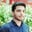Related Tags

vector assignment
vectors
r programming
communitycreator

# What are vectors and how to do vector assignment in R?Salman Yousaf

### Vectors

Vectors data structures in R consist of order collection of numbers, characters, or strings. There are two major types of vectors:

• Atomic or homogeneous vectors
• Recursive or heterogeneous vectors.

To create a vector named x, with values namely 10, 20, 30, 3.5 and 3.8, use c() function in R command:

x <- c(10, 20, 30, 3.5, 3.8)


Note: The c() function will return a vector.

### Assignment in R

There are various methods or operators in R to perform assignment operations. Let’s discuss some techniques to perform this operation on vectors.

### Using<- operator

This assignment operator(<-) consists of two characters:

• < (less than)
• - (minus)

These two characters occur side by side, while the tip points to a variable will receive an assignment value. The assignment of <- operator is also possible in reverse direction ->.

### Example

Let’s look at the code below:

# USING <- OPERATOR
cat("Arrow assignment: ")
x <- c(10, NA, 30, 'shot', 3.8)
cat(x, end='\n')
# Assignment operator in reverse direction
c(10.4, 5.6, 3.1, 6.4, 'AB') -> y
cat("Revered arrow assignment: ", y, end='\n')

### Explanation

• Line 3: We create a vector using the c() function and assign it to the x variable using <- operator.
• Line 4: We print the vector x on console.
• Line 6: We create a vector using the c() function and assign it to y variable using -> reversed operator.
• Line 7: We print vector y to the console.

### Using = operator

Equal to (=) operator is also used for value assignment. So, we can perform the above operation like:

x = c(9, 8, 23, 4.5, 9.8)


### Example

Let’s understand the equal = operator with the help of a code example:

# USING = OPERATOR
x = c(10, NA, 30, 'shot', 3.8)
cat(x)

### Explanation

• Line 2: We create a vector x using the c() function and equal to = operator.
• Line 3: We print x to the console.

### Using assign()

Assignment can also be made by using the assign() method. It’s an equivalent method of assignment as above.

### Example

In the example below, we use the assign() function in detail:

# USING assign() FUNCTION
assign("x", c(10, NA, 30, 'Edpresso', 3.8))
cat(x)

### Explanation

• Line 2: We use the assign() function for assignment second argument value to first one.
• Line 3: We print the vector x to the console.

RELATED TAGS

vector assignment
vectors
r programming
communitycreator

CONTRIBUTORSalman Yousaf
RELATED COURSES

View all Courses

Keep Exploring

Learn in-demand tech skills in half the time